python创意小作品代码-超全代码详解 Python 制作精美炫酷图表教程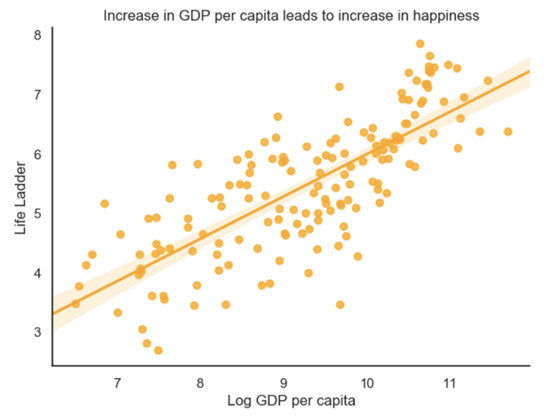《世界幸福报告》试图回答世界范围内影响幸福的因素。· 我使用Python进行绘图的经历

· 分布的重要性

· 加载数据和包导入

· 迅速：使用Pandas进行基本绘图

· 美观：使用Seaborn进行高级绘图

· 精彩：用plotly创造精彩的互动情节

1. 我使用Python进行绘图的经历Matplotlib

Seaborn

Bokeh

Plotly

Kepler.gl (地理空间数据优秀奖)

Kepler.gl不是一个Python库，而是一款强大的基于web的地理空间数据可视化工具。只需要CSV文件，就可以使用Python轻松地创建文件。试试吧!

2. 分布的重要性3. 加载数据和包导入# Loadthe datadata= pd.read_csv( https://raw.githubusercontent.com/FBosler/AdvancedPlotting/master/combined_set.csv)# thisassigns labels per yeardata[ Mean Log GDP per capita] = data.groupby( Year)[ Log GDP per capita].transform(pd.qcut,q= 5,labels=([ Lowest, Low, Medium, High, Highest]))

· 年份：计量年（2007 -2018）

· 人均GDP：根据世界银行2018年11月14日发布的《世界发展指标》（WDI），将人均GDP调整为PPP（2011年不变价国际元）

· 社会支持：对下面问题的回答："遇到困难时，是否可以随时获得亲戚或朋友的帮助？”

· 出生时预期健康寿命：出生时预期健康寿命是根据世界卫生组织（WHO）全球卫生观察站（GHO）数据库构建的，数据分别来自2005年、2010年、2015年和2016年。

· 自由选择权：回答下面这个问题："你是否对自己生活的选择自由感到满意？”

· 慷慨：对"过去一个月是否给慈善机构捐过款?”与人均GDP相比

· 政治清廉：回答"腐败现象在政府中是否普遍？”"腐败在企业内部是否普遍？”

· 积极影响：包括前一天快乐、欢笑和享受的平均频率。

· 负面影响：包括前一天焦虑、悲伤和愤怒的平均频率。

· 对国家政府的信心：不言自明

· 民主质量：一个国家的民主程度

· 执行质量：一个国家的政策执行情况

· Gapminder预期寿命：Gapminder的预期寿命

· Gapminder人口: 国家人口

importplotlyimport pandas aspdimport numpy asnpimport seaborn assnsimport plotly.express aspximport matplotlib%matplotlib inlineassertmatplotlib.__version__ == "3.1.0", """Please install matplotlib version 3.1.0 by running:1) !pip uninstall matplotlib2) !pip install matplotlib==3.1.0"""

4. 迅速：使用Pandas进行基本绘图Pandas有内置的绘图功能，可以在Series或DataFrame上调用。之所以喜欢这些绘图函数，是因为它们简洁、使用合理的智能默认值、很快就能给出进展程度。

np.exp(data[ data[ Year]==2018][ LogGDP per capita ]).plot(kind= hist)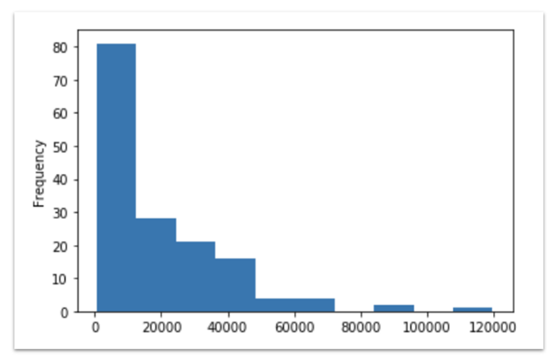2018年：人均GDP直方图。大多数国家都很穷，这一点也不奇怪！

· kind：Pandas必须知道需要创建什么样的图，可选的有以下几种：直方图（hist），条形图（bar），水平条图（barh），散点图（scatter），面积（area），核密度估计（kde），折线图（line），方框（box），六边形（hexbin），饼状图（pie）。

· figsize：允许6英寸宽和4英寸高的默认输出尺寸。需要一个元组（例如，我就经常使用figsize=(12,8)）

· title：为图表添加一个标题。大多数情况下，可以用这个标题来标明图表中所显示的内容，这样回过头来看的时候，就能很快识别出表的内容。title需要一个字符串。

· bins:直方图的bin宽度。bin需要一个值的列表或类似列表序列（例如， bins=np.arange(2,8,0.25)）

· xlim/ylim: 轴的最大和最小默认值。xlim和ylim都最好有一个元组（例如， xlim=(0,5)）

data[data[ Year] == 2018].set_index( Country name)[ Life Ladder].nlargest( 15).plot(kind= bar,figsize=( 12, 8))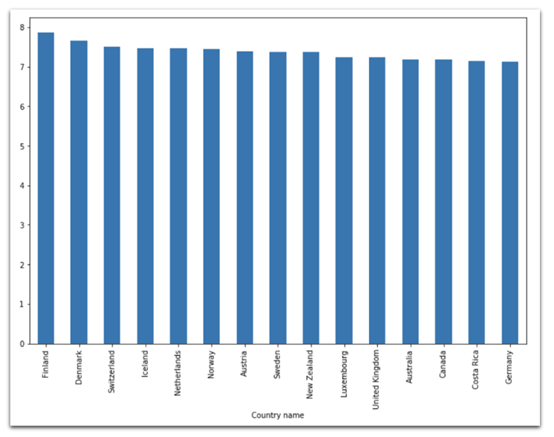2018年：芬兰位居15个最幸福国家之首

np.exp( data[data[ Year] == 2018].groupby( Continent)[ Log GDP per capita].mean).sort_values.plot(kind= barh,figsize=( 12, 8))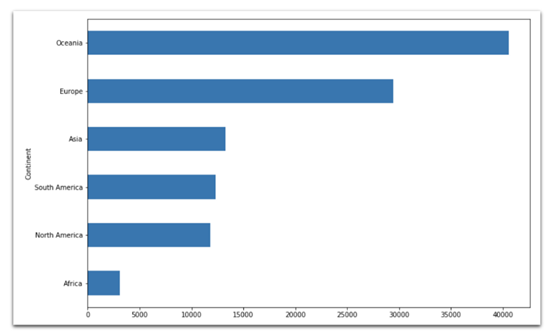data[ Life Ladder].plot(kind= box,figsize=( 12, 8))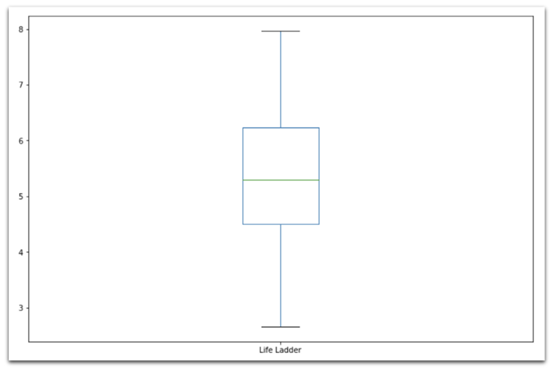data[[ Healthy life expectancyat birth, Gapminder Life Expectancy]].plot(kind= scatter,x= Healthy life expectancy at birth,y= Gapminder Life Expectancy,figsize=( 12, 8))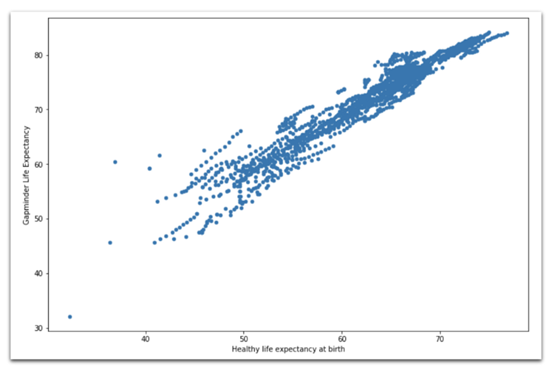Hexbin图

data[data[ Year ] == 2018].plot( kind= hexbin , x= Healthy life expectancy at birth , y= Generosity , C= Life Ladder , gridsize=20, figsize=(12,8), cmap="Blues", # defaults togreenish sharex=False # required to get rid ofa bug)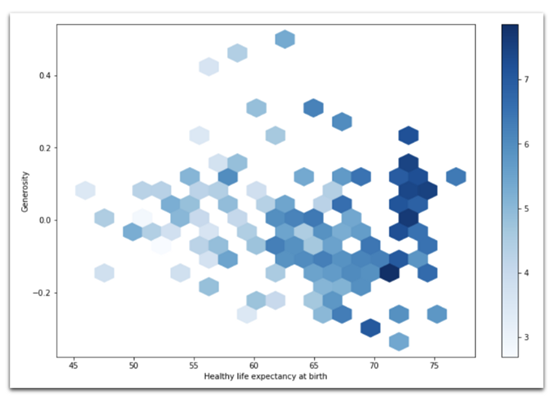2018年：Hexbin图，表示人的平均寿命与慷慨程度之间的关系。格子的颜色表示每个格子的平均寿命。

data[ data[ Year] == 2018].groupby([ Continent])[ Gapminder Population].sum.plot(kind= pie,figsize=( 12, 8),cmap= "Blues_r", # defaultsto orangish)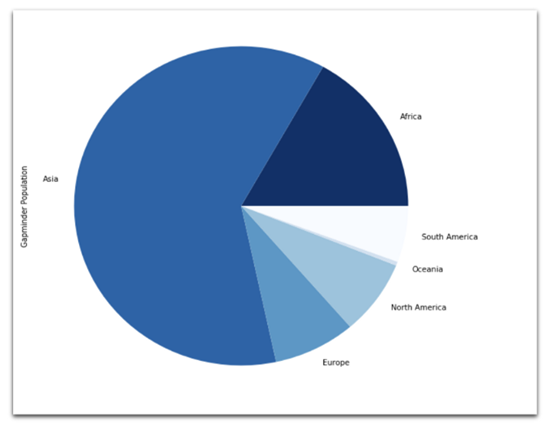2018年：按大洲划分的总人口数饼状图

data.groupby([ Year, Continent])[ Gapminder Population].sum.unstack.plot(kind= area,figsize=(12,8),cmap= "Blues", # defaults toorangish)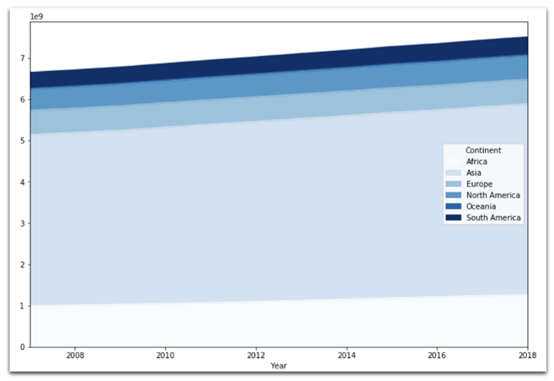data[ data[ Country name ] == Germany ].set_index( Year )[ Life Ladder ].plot( kind= line , figsize=(12,8))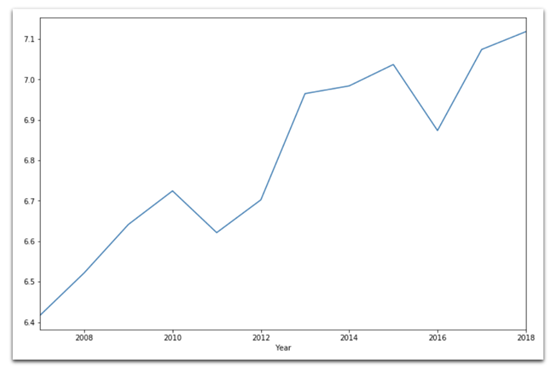5. 美观：使用Seaborn进行高级绘图

Seaborn使用的是默认绘图。要确保运行结果与本文一致，请运行以下命令。

sns.reset_defaultssns. set(rc={ figure.figsize:( 7, 5)},style= "white"# nicerlayout)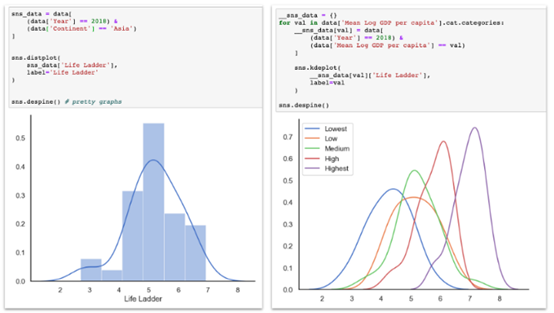sns.jointplot(x= Log GDP per capita,y= Life Ladder,data= data,kind= scatter# or kdeor hex)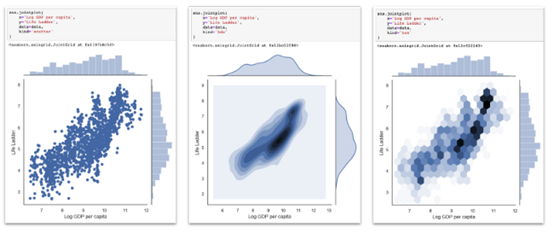Seaborn双标图，散点图、二元KDE和Hexbin图都在中心图中，边缘分布在中心图的左侧和顶部。

sns.scatterplot(x= Log GDP per capita,y= Life Ladder,data=data[data[ Year] == 2018],hue= Continent,size= Gapminder Population) # both, hue and size are optionalsns.despine # prettier layout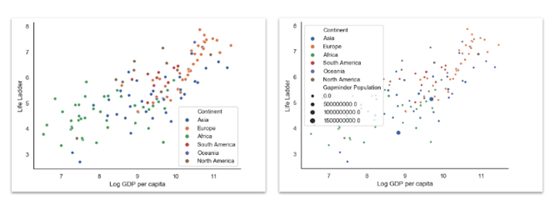sns.set( rc={ figure.figsize :(18,6)}, style="white")sns.violinplot( x= Continent , y= Life Ladder , hue= Mean Log GDP per capita , data=data)sns.despine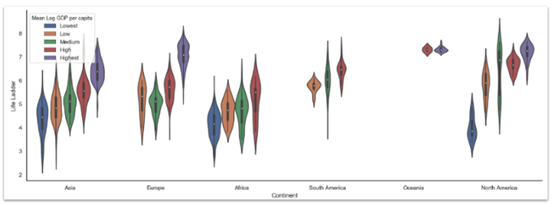Seaborn配对图是在一个大网格中绘制双变量散点图的所有组合。我通常觉得这有点信息过载，但它有助于发现规律。

sns. set(style= "white",palette= "muted",color_codes=True)sns.pairplot(data[ data.Year == 2018][[Life Ladder, Log GDP percapita,Social support, Healthy lifeexpectancy at birth,Freedom to make lifechoices, Generosity,Perceptions of corruption, Positive affect,Negative affect, Confidence innational government,Mean Log GDP per capita]].dropna,hue= Mean Log GDP per capita)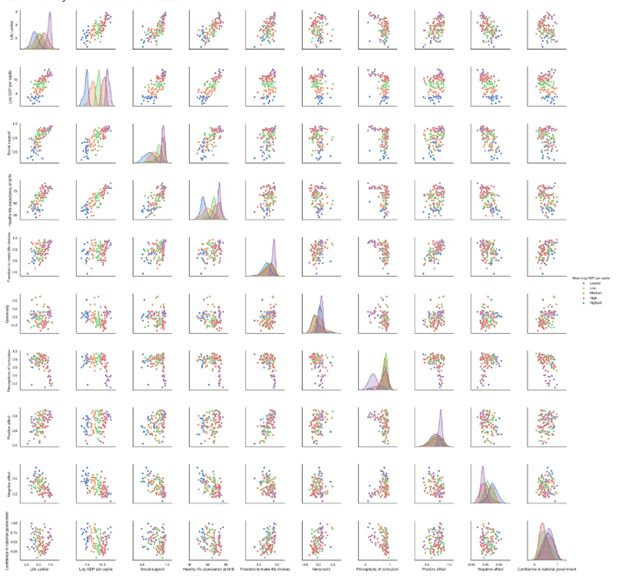Seaborn散点图网格中，所有选定的变量都分散在网格的下半部分和上半部分，对角线包含Kde图。

FacetGrids

FacetGrid— 折线图

g= sns.FacetGrid(data.groupby([ Mean Log GDP percapita, Year, Continent])[ Life Ladder].mean.reset_index,row= Mean Log GDP per capita,col= Continent,margin_titles=True)g = (g.map(plt.plot, Year, Life Ladder))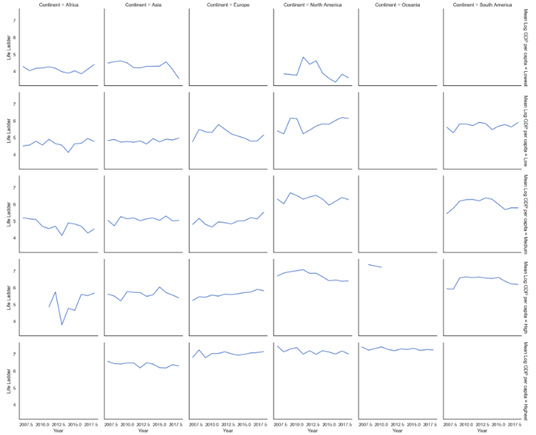y轴代表生活阶梯，x轴代表年份。网格的列代表大洲，网格的行代表不同水平的人均GDP。总体而言，北美人均GDP平均值较低的国家和欧洲人均GDP平均值中等或较高的国家，情况似乎有所好转

FacetGrid— 直方图

g= sns.FacetGrid(data,col= "Continent", col_wrap= 3,height= 4)g= (g.map(plt.hist, "Life Ladder",bins=np.arange( 2, 9, 0.5)))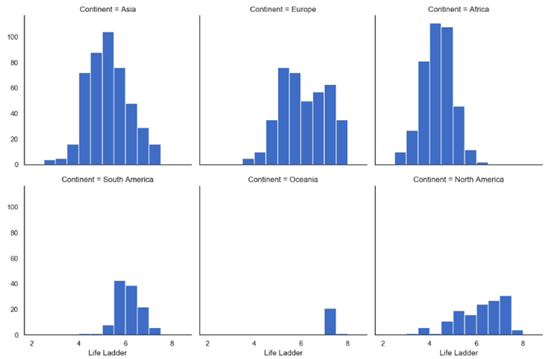FacetGrid— 带注释的KDE图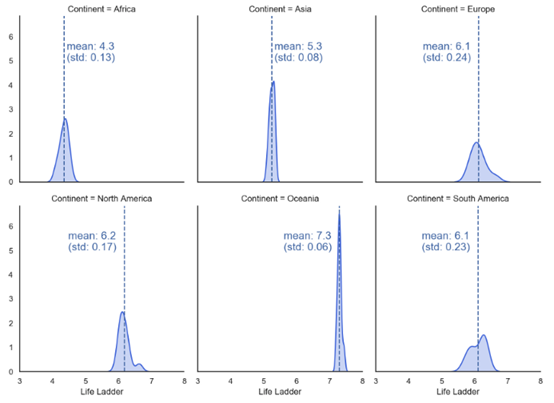annotate_facet_grid.py hostedwith ? by GitHub

FacetGrid— 热图Facet热图，外层的行显示在一年内，外层的列显示人均GDP，内层的行显示政治清廉，内层的列显示大洲。我们看到幸福指数朝着右上方向增加（即，高人均GDP和高政治清廉）。时间的影响还不确定，一些大洲（欧洲和北美）似乎比其他大洲（非洲）更幸福。

heatmap_facetgrid.py

defdraw_heatmap(data,inner_row, inner_col, outer_row, outer_col, values, vmin,vmax):sns.set(font_scale= 1)fg = sns.FacetGrid(data,row=outer_row,col=outer_col,margin_titles= True)position= left, bottom, width, height = 1.4, .2, .1, .6cbar_ax = fg.fig.add_axes( position)fg.map_dataframe(draw_heatmap_facet,x_col=inner_col,y_col=inner_row,values= values,cbar_ax=cbar_ax,vmin=vmin,vmax=vmax)fg.fig.subplots_adjust( right= 1.3)plt.showdefdraw_heatmap_facet(*args, **kwargs):data= kwargs.pop( data)x_col = kwargs.pop( x_col)y_col = kwargs.pop( y_col)values= kwargs.pop( values)d = data.pivot( index=y_col, columns=x_col, values= values)annot = round(d, 4).valuescmap = sns.color_palette( "Blues", 30) + sns.color_palette( "Blues", 30)[ 0:: 2]#cmap = sns.color_palette("Blues",30)sns.heatmap(d,**kwargs,annot=annot,center= 0,cmap=cmap,linewidth= .5)# Data preparation_ = data.copy_[ Year] = pd.cut(_[ Year],bins=[ 2006, 2008, 2012, 2018])_[ GDP per Capita] = _.groupby([ Continent, Year])[ Log GDP per capita].transform(pd.qcut,q= 3,labels=([ Low, Medium, High])).fillna( Low)_[ Corruption] = _.groupby([ Continent, GDP per Capita])[ Perceptions of corruption].transform(pd.qcut,q= 3,labels=([ Low, Medium, High]))_ = _[_[ Continent] != Oceania].groupby([ Year, Continent, GDP per Capita, Corruption])[ Life Ladder].mean.reset_index_[ Life Ladder] = _[ Life Ladder].fillna( -10)draw_heatmap(data=_,outer_row= Corruption,outer_col= GDP per Capita,inner_row= Year,inner_col= Continent,values= Life Ladder,vmin= 3,vmax= 8,)

heatmap_facetgrid.py hostedwith ? by GitHub

6. 精彩：用plotly创造精彩的互动情节· 悬停：当鼠标悬停在图表上时，会弹出注释

· 交互性：不需要任何额外设置，图表就可以进行交互（例如，一次穿越时间的旅程）

· 漂亮的地理空间图：Plotly已经内置了一些基本的映射功能，另外，还可以使用mapbox集成来制作令人惊叹的图表。

fig= x.(PARAMS)然后是 fig.show ，像这样：fig= px.scatter(data_frame= data[data[ Year ] ==2018],x= "Log GDP per capita",y= "Life Ladder",size= "GapminderPopulation",color= "Continent",hover_name= "Country name",size_max= 60)fig.show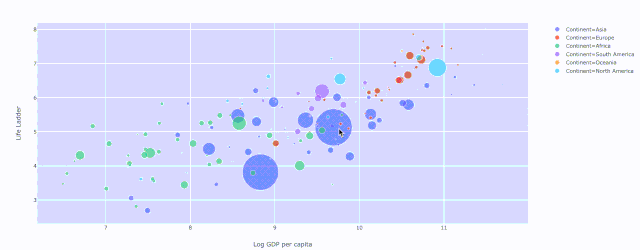Plotly散点图，绘制人均 GDP与生活阶梯的关系，其中颜色表示大洲和人口的大小

fig= px.scatter(data= data,x= "Log GDP per capita",y= "Life Ladder",animation_frame= "Year",animation_group= "Countryname",size= "GapminderPopulation",color= "Continent",hover_name= "Country name",facet_col= "Continent",size_max= 45,category_orders= { Year :list(range(2007,2019))})fig.show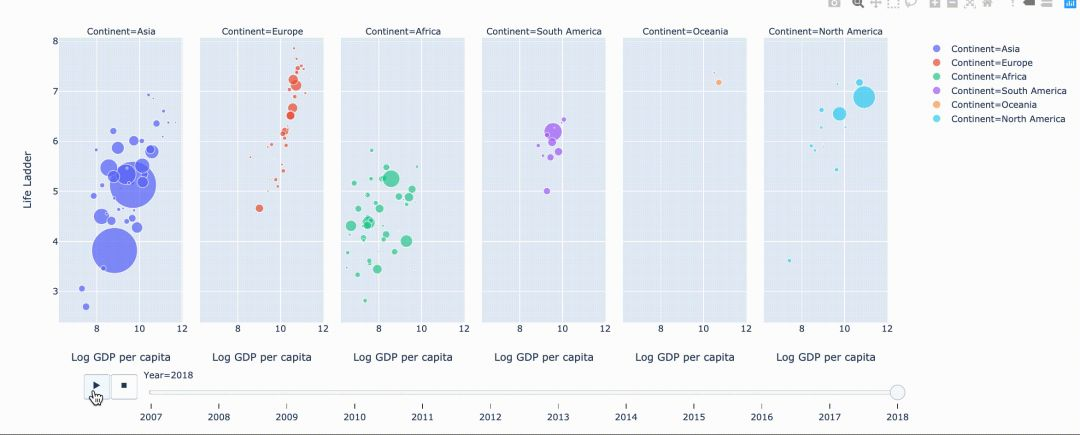defq_bin_in_3(col):returnpd.qcut(col,q= 3,labels=[ Low, Medium, High])_ = data.copy_[ Social support] = _.groupby( Year)[ Socialsupport].transform(q_bin_in_3)_[ Life Expectancy] =_.groupby( Year)[ Healthy life expectancy atbirth].transform(q_bin_in_3)_[ Generosity] =_.groupby( Year)[ Generosity].transform(q_bin_in_3)_[ Perceptions ofcorruption] = _.groupby( Year)[ Perceptions ofcorruption].transform(q_bin_in_3)_ = _.groupby([ Social support, LifeExpectancy, Generosity, Perceptions of corruption])[ LifeLadder].mean.reset_indexfig = px.parallel_categories(_, color= "LifeLadder", color_continuous_scale=px.colors.sequential.Inferno)fig.showfig = px.bar(data,x= "Continent",y= "Gapminder Population",color= "Mean Log GDP percapita",barmode= "stack",facet_col= "Year",category_orders={ "Year":range(2007,2019)},hover_name= Country name ,hover_data=["Mean Log GDP percapita","Gapminder Population","Life Ladder"])fig.show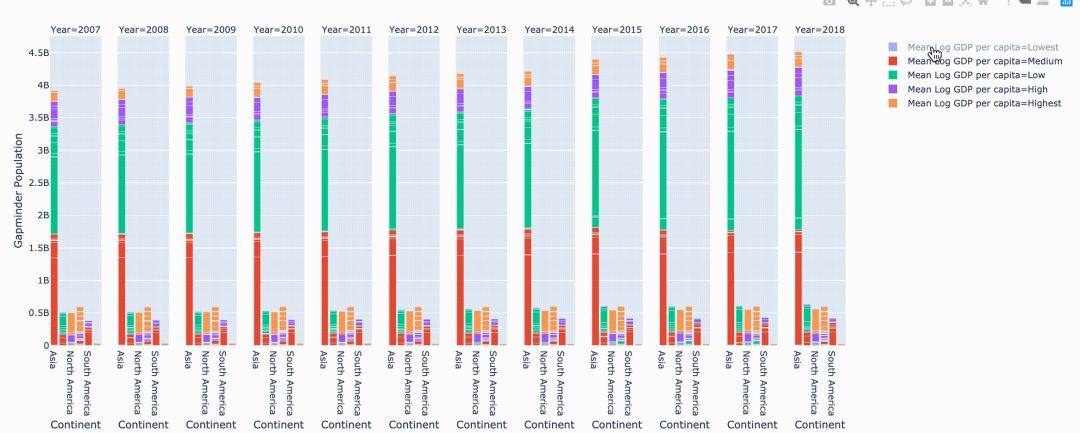fig = px.choropleth( data, locations="ISO3", color="Life Ladder", hover_name="Country name", animation_frame="Year")fig.show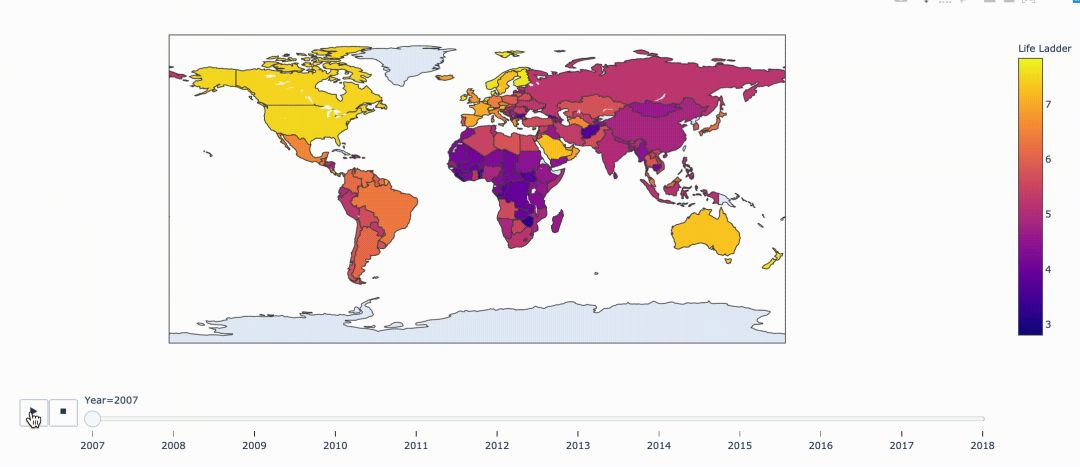06-3011-25429
06-238238
10-282995
10-30197
01-06
03-041万+
11-25143
11-201596
10-283080
01-28286
10-28437
11-291612
05-11
05-12
09-19
04-06点击重新获取扫码支付余额充值